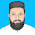It is a simple C# program to find Square and Square root of any number.
Here first text box will accept number to find square and square root. After typing a number, to find square of given number, click on ‘Square’ button, then you will get square in second text box. Click on ‘Square root’ button to get square root of given number.

Interface with Example Input – Output:

Code behind interface:

 using System; using System.Collections.Generic; using System.ComponentModel; using System.Data; using System.Drawing; using System.Linq; using System.Text; using System.Windows.Forms; namespace squar_and_squar_root {     public partial class Form1 : Form     {         double num;         public Form1()         {             InitializeComponent();         }                     private void Square_button_Click(object sender, EventArgs e)         {             num = Convert.ToDouble(number_box.Text);             result_text_box.Text = (num * num).ToString();         }         private void Square_root_button_Click(object sender, EventArgs e)         {             num = Convert.ToDouble(number_box.Text);             result_text_box.Text = (Math.Sqrt(num)).ToString();         }     } }

More C# programs here

1 comment:
1.Good Job For Beginners
Thank You Buddy